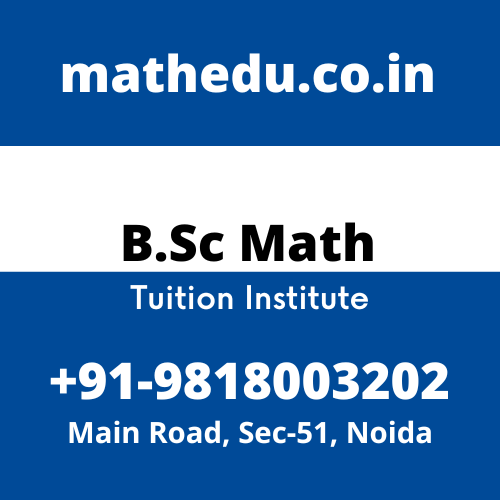# Real Analysis Tuition Class In Noida

### Real Analysis Tuition Class In NoidaAre You Looking for Real Analysis Tuition Class In Noida? Math Education Is a Live Tutoring Sites for The Best B.Sc Mathematics Tuition In Noida, Engineering Diploma, BBA, BCom. Highly Qualified Faculties Available to Teach University Students with Mathematics Subjects. Call Now +91-9818003202 For the Best Real Analysis Tuition Class In Noida.

## Course Objectives Of Real Analysis

The course will develop a deep and rigorous understanding of real line and of defining terms to prove the results about convergence and divergence of sequences and series of real numbers. These concepts has vide range of applications in real life scenario. Hence, Join The Best Online Math Tuition Class In Noida.

### Course Learning Outcomes: This course will enable the students to:

i) Understand many properties of the real line and learn to define sequence in terms of functions from to a subset of.
ii) Recognize bounded, convergent, divergent, Cauchy and monotonic sequences and to calculate their limit superior, limit inferior, and the limit of a bounded sequence.
iii) Apply the ratio, root, alternating series and limit comparison tests for convergence and absolute convergence of an infinite series of real numbers.

## Real Number System Tuition In Noida

Algebraic and order properties of R, Absolute value of a real number; Bounded above and bounded below sets, Supremum and infimum of a nonempty subset of R. Hence, Join The Best real Number System Tuition Classes.

## Properties of Real Number Tuition In Noida

The completeness property of Real Number, Archimedean property, Density of rational numbers in Real Number; Definition and types of intervals, Nested intervals property; Neighborhood of a point in Real Number, Open and closed sets in Real Number. Hence, Join The best Online Math Tutor In Noida.

## Sequences in Real Number Tuition In Noida

Convergent sequence, Limit of a sequence, Bounded sequence, Limit theorems, Monotone sequences, Monotone convergence theorem, Subsequences, Bolzano-Weierstrass theorem for sequences, Limit superior and limit inferior for bounded sequence, Cauchy sequence, Cauchy’s convergence criterion. Hence, Join The Sequences In Real Number Tuition In Noida.

## Infinite Series Tuition In Noida

Convergence and divergence of infinite series of real numbers, Necessary condition for convergence, Cauchy criterion for convergence; Tests for convergence of positive term series: Integral test, Basic comparison test, Limit comparison test, D’Alembert’s ratio test, Cauchy’s nth root test; Alternating series, Leibniz test, Absolute and conditional convergence. Hence, Join The Infinite Series Tuition In Noida.

Hello 👋